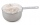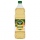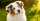# Mixed fractions calculator

The calculator performs basic and advanced operations with mixed numbers, fractions, integers, decimals. Mixed fractions are also called mixed numbers. A mixed fraction is a whole number and a proper fraction combined, i.e. one and three-quarters. The calculator evaluates the expression or solves the equation with step-by-step calculation progress information. Solve problems with two or more mixed numbers fractions in one expression.

## Result:

### 16/3 = 16/3 = 5 1/3 ≅ 5.3333333

Spelled result in words is five and one third (or sixteen thirds).

#### Examples:

sum of two mixed numbers: 1 3/4 + 2 3/8
addition of three mixed numbers: 1 3/8 + 6 11/13 + 5 7/8
addition of two mixed numbers: 2 1/2 + 4 2/3
subtracting two mixed numbers: 7 1/2 - 5 3/4
multiplication of mixed numbers: 3 3/4 * 2 2/5
comparing mixed numbers: 3 1/4 2 1/3
changing improper fraction to mixed number: 9/4
What is 3/4 as a mixed number: 3/4
subtracting mixed number and fraction: 1 3/5 - 5/6
sum mixed number and an improper fraction: 1 3/5 + 11/5

## Mixed fractions in word problems:

• A more than bWhat is 5 1/7 more than 2 3/7?
• Mixed2improperWrite the mixed number as an improper fraction. 166 2/3
• Zailene 2Zailene has 8 2/3 cup of flour. She used 5 1/2 cups of flour. How many cups of flour was left to her?
• A scienceA science experiment calls for making 3 and 2/3 cups of distilled water with 1 and 3/5 cups of vinegar and 2/3 cups of liquid detergent how much liquid in all and cups is needed?Add this two mixed numbers: 1 5/6 + 2 2/11=
• Difference mixed fractionsWhat is the difference between 4 2/3 and 3 1/6?
• ZaileneZailene wants to make two kinds of kakanin, puto and kutsina. Puto recipe needs 3 2/5 cup of flour while Kutsinta needs 5 ¼ cup of flour. How many cups of flour will Zailene be needed in all?
• Cups of punchCyka made 6 19/20 cups of punch punch at two different types of juice in it. If the punch had 4 1/5 cups of one type of juice how many cups of the other type of juice did it have?
• A fullA full bag of dog food weights 5 1/2 pounds. How much dog food do you have if there are 3 3/4 bags in the cupboard?
• The product 3The product of two numbers is 15 ⁵/₆. If one of the numbers is 5 ²/₃, find the other.3 3/4 + 2 3/5 + 5 1/2 Show your solution.Bill was riding his bike on a bike path that was 5 1/4 miles long. He rode his bike awhile and stopped for s drink or water. Then he rode 2 7/10 miles and was at the end of the path. How far had Bill ridden before he stopped for water?The first team member in a 926-person relay race must run 2 1/4 laps, the second team member must run 1 1/2 laps, and the third team member must run 3 1/4 laps. How many laps in all must each team run?Sum of the two numbers is 15 1/6. If one of the numbers is 2 2/3, find the other number.Maya bought 18 apples. She used 50% of the apples to make apple pies and 1/3 of the apples was mixed with other fruits to make salad. How many apples were left?Find the perimeter of the rectangle. A rectangle with a base of (3 5/6) m and a height of (2 3/7) m Its perimeter is x= meters. (Use fraction in your answer. )Add this two mixed numbers: 1 5/6 + 2 2/11=